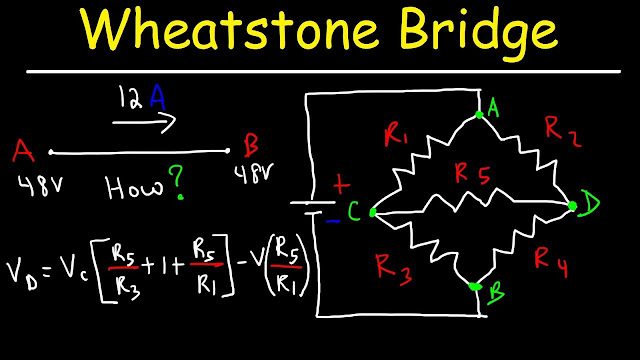How To Solve The Wheatstone Bridge Circuit

# How To Solve The Wheatstone Bridge CircuitHow To Solve The Wheatstone Bridge Circuit

This video tutorial explains how to solve the WheatStone Bridge Circuit both in the balanced and unbalanced condition.  It explains how to calculate the current in each resistor, the voltage across the resistors, as well as the equivalent resistance of the circuit.  This video also explains how to solve the wheatstone bridge circuit using Kirchoff's current laws.  It also provides formulas that can help you to quickly get the answer.
Related posts: